How many valence electrons are in an atom of sulfur?Hali

Concept:

Step:1 The distribution of electrons in different orbitals is called electronic configuration. The electrons filled in the outermost shell or the electrons present in the highest principal energy level are called valence electrons.

Step: 2 The filling of electrons in different energy levels or orbitals is done in accordance to Aufbau principle. It states that electrons are filled in the orbitals in order of increasing energy of the orbitals. The increasing order of energy of the orbitals is as follows:

Solution:

Step:1 The atomic number of sulfur is 16. The number of electrons in sulfur is 16. The electronic configuration of sulfur is as follows: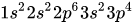Step: 2 The highest principal energy level is 3. The electrons present in 2s and 2p orbital are valence electrons. Distribution of electrons in different orbitals in sulfur atom is shown as: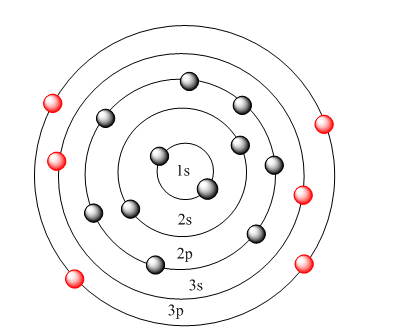Therefore, the number of valence electrons in an atom of sulfur is 6.

Similar problems:

Question:

1. How many valence electrons are in atom of phosphorous?

Solution:

Step: 1 The atomic number of phosphorous is 15. The number of electrons in phosphorous is 15. The electronic configuration of phosphorous is as follows: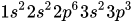Step: 2 The highest principal energy level is 3. The electrons present in 3s and 3p orbital are valence electrons. Distribution of electrons in different orbitals in the phosphorous atom is shown as: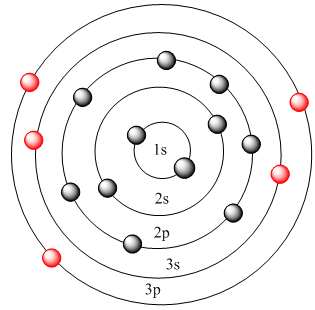Therefore, the number of valence electrons in an atom of phosphorous is 5.

Question:

2. How many valence electrons are in an atom of oxygen?

Solution:

Step: 1 The atomic number of oxygen is 8. The number of electrons in oxygen is 8. The electronic configuration of oxygen is as follows: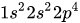Step: 2 The highest principal energy level is 2. The electrons present in 2s and 2p orbital are valence electrons. Distribution of electrons in different orbitals in oxygen atom is shown as: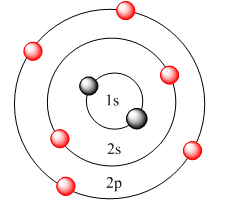Therefore, the number of valence electrons in an atom of oxygen is 6.

Need homework help now?

tutors online

Related Questions
2. (11 marks) a) At 400 °C, ammonium iodide decomposes in the following endothermic reaction: NH41(s) + NH3(g) + HI(g) Kp = 0.215 at 400 °C. Write the equilibrium expression for this reaction. 15.0 g of NH4I was placed in an empty 3.0 L flask and heated to 400 °C. What is the partial pressure of NH3 in the flask once the reaction has reached equilibrium? How would the following changes to the equilibrium mixture affect the number of moles of NH3 in the flask and the value of K, once equilibrium is re-established? (Use increase, decrease or stay the same.) Change Effect on moles of NH3 Ke value Increase temperature Increase volume of flask Remove half of HI gas
Notes
Me

OR

Don't have an account?

Join OneClass

Access over 10 million pages of study
documents for 1.3 million courses.

Join to view

OR

By registering, I agree to the Terms and Privacy Policies
Just a few more details

So we can recommend you notes for your school.